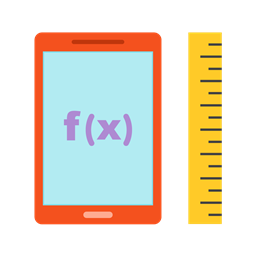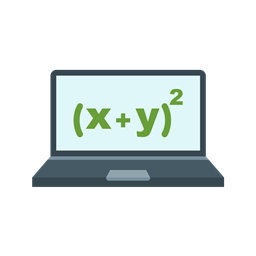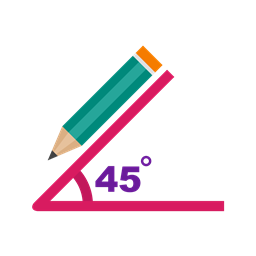# Math Summer Bootcamps

### About our Math Summer Bootcamps

##### Pre-Algebra 1

Pre-Algebra 1 is aimed at giving students a solid foundation as they embark upon the study of Algebra. This camp is ideal for students in advanced elementary and middle school levels (grades 5-7). It begins with a review of Arithmetic and slowly expands the student’s vocabulary to new types of numbers (negatives, fractions, decimals) explaining how to reason in these number systems. With the improved skills in abstraction, the camp helps them understand how to formulate and solve linear equations in one variable.

##### Pre-Algebra 2

Pre-Algebra 2 builds offf from where Kodeclik’s Pre-Algebra 1 left and introduces advanced concepts from factorization and basic number theory. It also introduces many applications including making sense of data, probability, and understanding trends and patterns. The key skill imparted by this camp is an introduction to a range of problem solving strategies that will get regularly reinforced with each topic. This camp prepares students for a detailed study of Algebra.

##### Geometry 1

Geometry 1 begins from the most basic ingredients of geometry such as points, lines, and angles and uses triangles and quadrilaterals as the medium to introduce a range of geometric notions such as symmetry and congruence. Students are also introduced to studying these notions in the two-dimensional cartesian coordinate system as well as computing area and perimeter of geometric shapes. This camp places a heavy emphasis on developing the student’s geometric intuition via visualizations and drawings before the student embarks on more rigorous proof-based geometry camps.

##### Geometry 2

The Geometry 2 camp introduces more complex shapes (e.g., circles) and three dimensional objects (e.g., cuboids, cylinders, cones, spheres). It presents a unifying emphasis on transformations to relate geometric shapes and, further, combines skills from Algebra and Geometry to introduce notions like the Pythagorean theorem and basic trigonometry. Students are also introduced to the importance of proofs in geometric reasoning.#### Pre Algebra 1

###### Students will begin with an Arithmetic review, and move on to the distributive property, order of precedence, fractions and ratios, speed and rates of variation, decimals, percentages, negative numbers, variables and simplification of expressions.#### Pre Algebra 2

###### Students will learn factors, exponents, squares and square roots, greatest common divisors and least common multiples, prime factorization, ratios and proportion, direct and inverse variation graphing data, basic combinatorics and probability.#### Geometry 1

###### Students will learn points, lines and angles, properties of triangles, quadrilaterals, special types of triangles, special types of quadrilaterals, similarity and congruence properties, area and perimeter, and the co-ordinate plane.